# 这样拟合正弦函数你会吗

## 1 动手实现线性回归

### 1.1 深度学习框架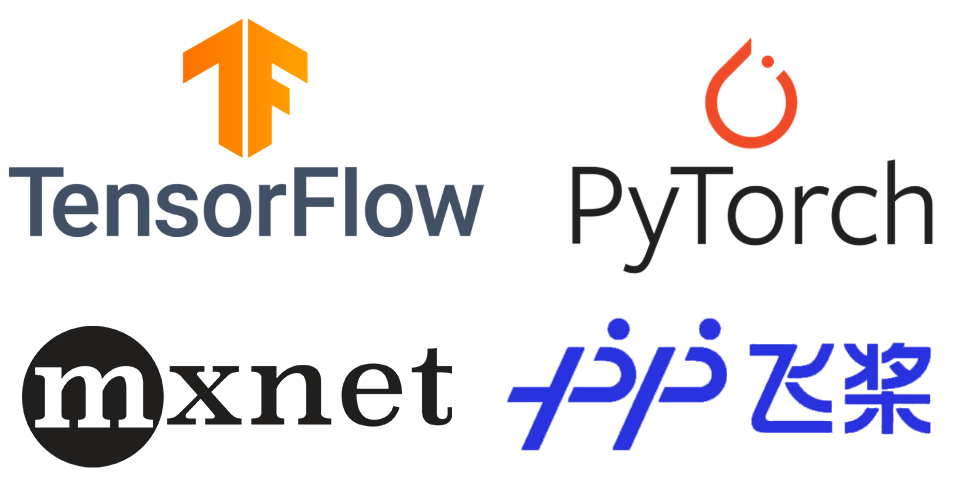### 1.2 安装Pytorch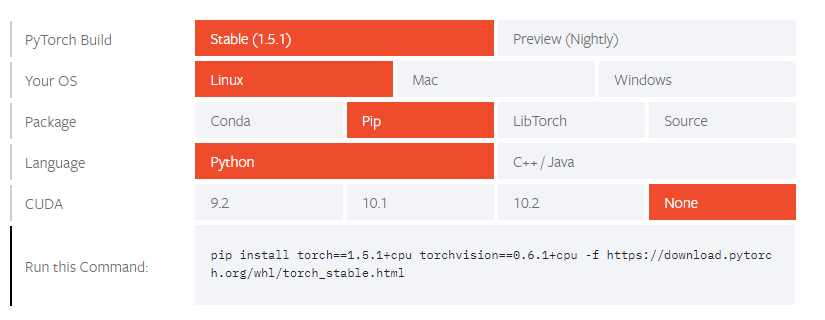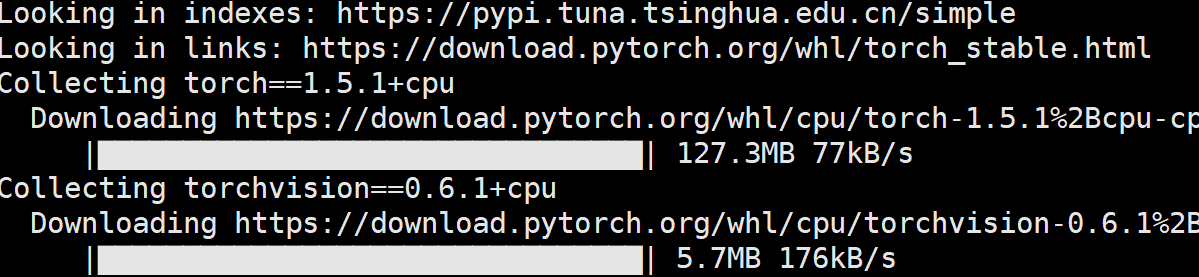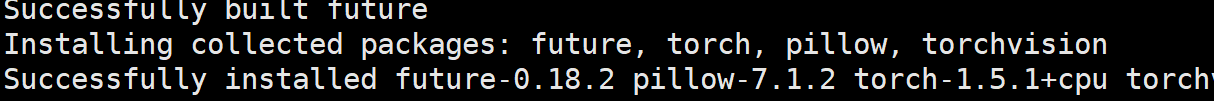### 1.3 线性回归的实现

• 导入相关包

这里首先导入我们需要用到的相关包，在本示例中一共有4个：

from sklearn.datasets import load_boston
from sklearn.preprocessing import StandardScaler
import numpy as np
import torch


前三个我们之前都用过就不再做介绍，第4个就是我们安装好的Pytorch框架。

• 载入数据

def load_data():
x, y = data.data, data.target
ss = StandardScaler()
x = ss.fit_transform(x)# 特征标准化
x = torch.tensor(x, dtype=torch.float32)
y = torch.tensor(y, dtype=torch.float32)
return x, y


上述代码中，前面5行我们之前也都用过；而第6，7行的作用就是将numpy类型的变量转化为Pytorch框架所接受的tensor张量。同时需要注意的是，在使用所有有关torch方法时，其所接收的变量都必须是<class 'torch.Tensor'>类型的，如果不是则需要通过torch.tensor()进行转换。

• 预测

def forward(x, weights, bias):  # 预测
y = torch.matmul(x, weights) + bias
return y


这几行代码与之前在机器学习中用numpy实现的并无太大差异，只是将np.matul变成了torch.matul。同时，在定义这个函数时我们并没有使用prediction这个名字，而是使用了forward这个词，具体含义我们后续再解释。

• 定义损失

def loss(y, y_hat):
return 0.5 * torch.mean((y - y_hat.reshape(y.shape)) ** 2)
# 一定要注意将两者reshape一样


这两句代码同样很简单，但是需要注意的是在做差的时候，要将两者转换成同一个shape不然可能触发广播机制，造成不易察觉的严重错误。例如形状为[3,1]张量减去形状为[1,3]的张量，得到的结果将是一个[3,3]形状的结果（反过来也是一样）。

• 定义评估标准

def RMSE(y, y_hat):


在这里，我们依旧选择RMSE作为评估标准，即损失开方即可。

• 定义梯度下降

def gradientDescent(params, lr):
for param in params:


其中前三行代码是执行参数的梯度下降步骤，需要注意的是由于在定义损失的时候计算的是MSE，也就是已经除以了样本总数，所有在第3行代码中param.grad就是每个样本的平局梯度不需要再除以样本数。同时，最后一行代码是将先前的梯度值清除。因为在Pytorch中，每次计算出来的梯度都会累积（accumulate）到一起，所有每一次梯度下降后都需要置为0。

• 训练模型

def train(x, y):
epoches = 100
lr = 0.3
w = torch.tensor(np.random.normal(0, 0.1, [x.shape, 1]), 			                              dtype=torch.float32, requires_grad=True)
for i in range(epoches):
logits = forward(x, w, b)
l = loss(y, logits)
l.backward()
if i % 5 == 0:
print("Epoch: {}, loss: {}".format(i, l))
logits = forward(x, w, b)
print("RMSE: {}".format(RMSE(y, logits)))


如上代码所示，其中第4、5行为初始化权重和截距，其原理就是将一个numpy类似的值转换为tensor，同时需要指定类型；最后requires_grad=True表示将该张量设置为一个可求导的状态，也就是说后面如果我们需要对某个变量求导，那么这个变量在定义的时候该属性就需要设置为True。从第6行代码开始就是执行训练的步骤，l=loss(y,logits)是用来计算整个目标函数的损失，而第7行代码l.backward()就是用来求解损失l关于所有变量的梯度。可以看到一行代码就能求解得到所有参数的梯度，这就是框架的作用。

同时，第10行代码就开始调用梯度下降算法。值得注意的是在函数gradientDescent()中，param其实就是wb，而param.data访问的就是param对应的值所进行的原地（in-place）操作，暂时不理解也无所谓，知道更新梯度是这么写的即可。

• 运行结果

Epoch: 0, loss: 307.88818359375
Epoch: 10, loss: 12.249261856079102
Epoch: 20, loss: 11.236662864685059
Epoch: 30, loss: 11.09315013885498
Epoch: 40, loss: 11.032454490661621
Epoch: 50, loss: 11.000597953796387
Epoch: 60, loss: 10.982012748718262
Epoch: 70, loss: 10.970415115356445
Epoch: 80, loss: 10.96288013458252
Epoch: 90, loss: 10.957874298095703
RMSE: 3.309760093688965


可以发现，随着迭代次数的增加，大约在100轮之后损失函数就开始收敛了。最终得到的RMSE指标为3.30。

## 2 动手实现正弦函数拟合

### 2.1 网络结构图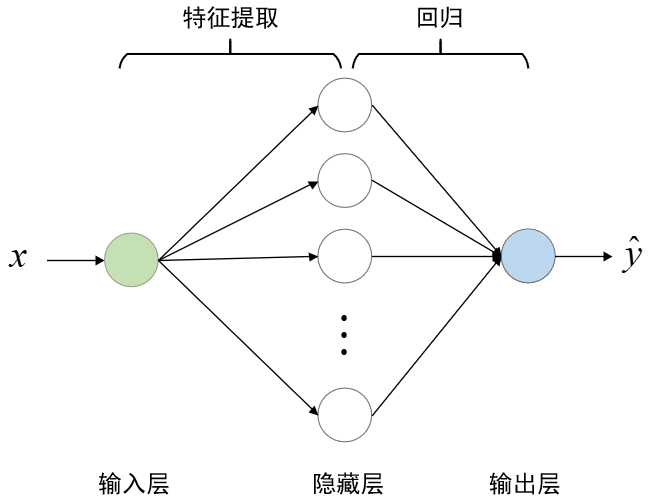### 2.2 拟合正弦函数

• 导入相关包

import torch
import numpy as np
import matplotlib.pyplot as plt


由于后期需要可视化，所以导入了matplotlib库。

• 构造数据集

def make_data():
num_samples = 200
x = torch.linspace(-np.pi, np.pi, num_samples,
dtype=torch.float32).reshape(-1, 1)
y = torch.sin(x)
return x, y


这里我们用torch.linspace()来构造区间 [ − π , π ] [-\pi,\pi] 之间的200个样本点。同时，损失函数和梯度下降部分同上面一样就不再赘述。

• 预测

def forward(x, w1, b1, w2, b2):
out1 = torch.matmul(x, w1) + b1
out1 = torch.sigmoid(out1)
out2 = torch.matmul(out1, w2) + b2
return out2


根据图5中的结构，我们能写出如上5行代码，其中第3行代码是对提取得到的特征进行非线性变换，第4行代码为进行回归预测。

• 训练

def train(x, y):
input_nodes = x.shape# 1
hidden_nodes = 50
output_nodes = 1
epoches = 3000
lr = 0.2
w1 = torch.tensor(np.random.normal(0, 0.5, [input_nodes, hidden_nodes]), dtype=torch.float32, requires_grad=True)
w2 = torch.tensor(np.random.normal(0, 0.5, [hidden_nodes, output_nodes]), dtype=torch.float32, requires_grad=True)
#.....
#.....


由于篇幅有限如上所示仅为部分的代码，完整部分请直接参见示例代码。从第3行代码可以看到我们将隐藏层神经元的个数设置为了50，后面也会对不同值的结果进行比较。

• 结果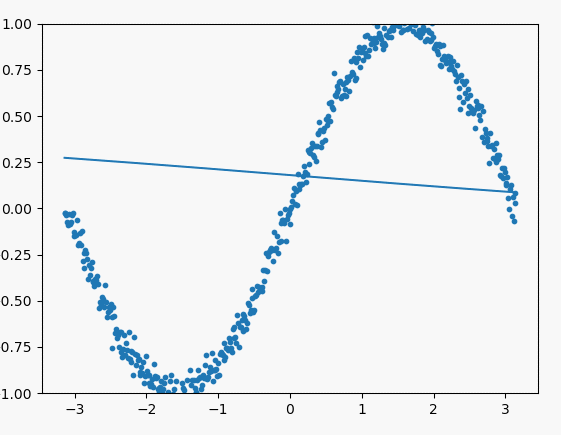### 2.3 对比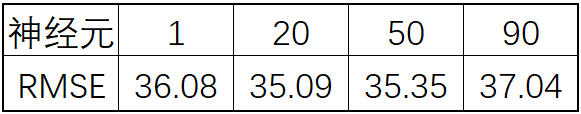## 3 总结

### 引用

深度学习与PyTorch入门实战教程 https://www.bilibili.com/video/BV11z4y1R748?p=39

Pytorch官网 https://pytorch.org/

示例代码：https://github.com/moon-hotel/DeepLearningWithMe

### 推荐阅读

你告诉我什么是深度学习10-14
01-07458202-253万+
05-044268
09-172730
11-233003
12-21718
05-179387
03-241214
04-045867
02-152873
06-071万+
09-083万+
03-125864
07-157976
01-292万+
04-252万+
07-151万+
03-098566
11-068030